SSC BOARD PAPERS IMPORTANT TOPICS COVERED FOR BOARD EXAM 2024

### Practice Set 6.2 Trigonometry Class 10th Mathematics Part 2 MHB Solution

Practice Set 6.2

1. A person is standing at a distance of 80m from a church looking at its top. The angle…
2. From the top of a lighthouse, an observer looking at a ship makes angle of depression…
3. Two buildings are facing each other on a road of width 12 metre. From the top of the…
4. Two poles of heights 18 metre and 7 metre are erected on a ground. The length of the…
5. A storm broke a tree and the treetop rested 20 m from the base of the tree, making an…
6. A kite is flying at a height of 60 m above the ground. The string attached to the kite…

###### Practice Set 6.2
Question 1.

A person is standing at a distance of 80m from a church looking at its top. The angle of elevation is of 45°. Find the height of the church.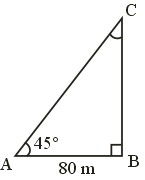Let 'A' be the person, standing 80 m away from a church BC,

Angle of elevation, ∠BAC = θ = 45°

Clearly, ∆ABC is a right-angled triangle, in which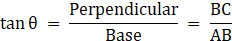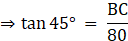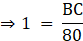⇒ BC = 80 m

Therefore, Height of church is 80 m.

Question 2.

From the top of a lighthouse, an observer looking at a ship makes angle of depression of 60°. If the height of the lighthouse is 90 metre, then find how far the ship is from the lighthouse. (√3 = 1.73)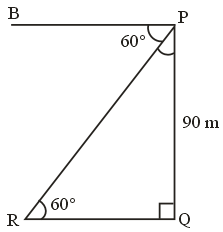Let PQ be a light house of height 80 cm such that PQ = 90 m

And R be a ship.

Angle of depression from P to ship R = ∠BPR = 60°

Also, ∠PRQ(say θ) = ∠BPR = 60° [Alternate Angles]

Clearly, PQR is a right-angled triangle.

Now, In ∆PQR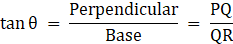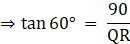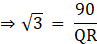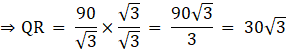⇒ QR = 30(1.73)

⇒ QR = 51.90 m

Hence, Ship is 51.90 m away from the light house.

Question 3.

Two buildings are facing each other on a road of width 12 metre. From the top of the first building, which is 10 metre high, the angle of elevation of the top of the second is found to be 60°. What is the height of the second building?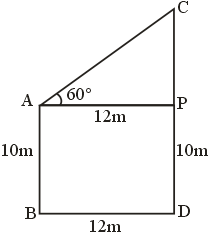Let AB and CD be two building, with

AB = 10 m

And angle of elevation from top of AB to top of CD = ∠CAP = 60°

Width of road = BD = 12 m

Clearly, ABDP is a rectangle

With

AB = PD = 10 m

BD = AP = 12 m

And APC is a right-angled triangle, In ∆APC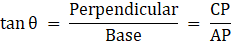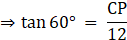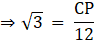⇒ CP = 12√3 m

Also,

CD = CP + PD = (12√3 + 10) m

Hence, height of other building is (10 + 12√3 m).

Question 4.

Two poles of heights 18 metre and 7 metre are erected on a ground. The length of the wire fastened at their tops in 22 metre. Find the angle made by the wire with the horizontal.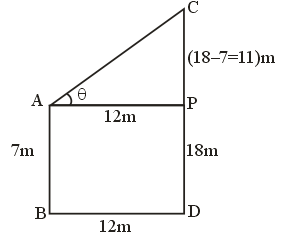Let AB and CD be two poles, and AC be the wire joining their top.

and

Angle made by wire with horizontal = ∠CAP = θ [say]

Given,

AB = 7 m [Let AB be smaller pole]

CD = 18 m

AC = 22 m

Clearly, APDB is a rectangle with

AB = PD = 7 m

Also,

CP = CD - PD = 18 - 7 = 11 m

In ∆APC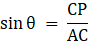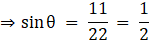⇒ θ = 30°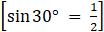Question 5.

A storm broke a tree and the treetop rested 20 m from the base of the tree, making an angle of 60° with the horizontal. Find the height of the tree.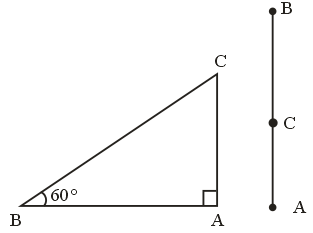Let AB be a tree, and C is the point of break.

Height of tree = BC + AC

As, the treetop is rested 20 m from the base, making an angle of 60° with the horizontal.

In ∆ABC

AB = 20 m

∠ABC, θ = 60°

Now,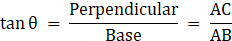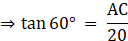⇒ AC = 20 tan 60°

⇒ AC = 20√3 m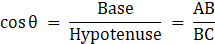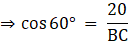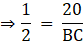⇒ BC = 40 m

Height of tree = BC + AC = (40 + 20√3) meters.

Question 6.

A kite is flying at a height of 60 m above the ground. The string attached to the kite is tied at the ground. It makes an angle of 60° with the ground. Assuming that the string is straight, find the length of the string. (√3 = 1.73 )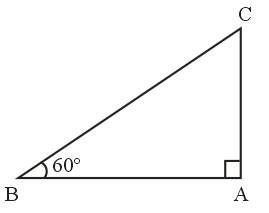Let AC be a string and kite is flying at point A, with height

AB = 60 m

Angle make by string with horizontal, θ = ∠ACB = 60°

Clearly, ABC is a right-angled triangle.

In ∆ABC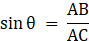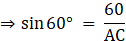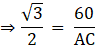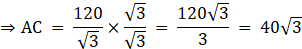⇒ AC = 40(1.73)

⇒ AC = 6.92 m

Hence, length of string is 6.92 m